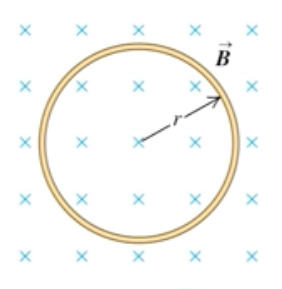Need Help?

Subscribe to Physics 2

###### \${selected_topic_name}
• Notes
• Comments & Questions

$\begin{array}{l}{\text { A circular loop of wire }} \\ {\text { with radius } r=0.0250 \mathrm{m} \text { and resist- }} \\ {\text { ance } R=0.390 \Omega \text { is in a region of spa- }} \\ {\text { tially uniform magnetic field, as shown }} \\ {\text { in Fig. } \mathrm{E} 29.21 . \text { The magnetic field is }} \\ {\text { directed into the plane of the figure. }}\end{array}$

$\begin{array}{l}{\text { At } t=0, B=0 . \text { The magnetic field }} \\ {\text { then begins increasing, with } B(t)=} \\ {\left(0.380 \mathrm{T} / \mathrm{s}^{3}\right) t^{3} . \text { What is the current in }} \\ {\text { the loop (magnitude and direction) at }} \\ {\text { the instant when } B=1.33 \mathrm{T} ?}\end{array}$$|\varepsilon|=\left|\frac{d \Phi_{B}}{d t}\right|$$=A\left|\frac{d B}{d t}\right|$

$=\pi r^{2}\left|\frac{d B}{d t}\right|$

$I=\frac{|\varepsilon|}{R}=$$\frac{\pi r^{2}}{R}\left|\frac{d B}{d t}\right|=\frac{\pi(0.008)^{2}}{0.16}(0.68)$

$=0.03076 \mathrm{A}$

$p=I^{2} R$$\rightarrow(0.03076)^{2}(0.16)=1.51 * 10^{-4} \mathrm{W}$

$|\varepsilon|=\left|\frac{d \Phi_{B}}{d t}\right|=$$=A\left|\frac{d B}{d t}\right|$

$=\pi r^{2}\left|\frac{d B}{d t}\right|$

$=\pi(0.025)^{2}\left[3 * 0.38 t^{2}\right]$

$=\left(2.238 * 10^{-3} \mathrm{V} / \mathrm{s}^{2}\right) \mathrm{t}^{2}$

$I=\frac{|\varepsilon|}{R}$$=\frac{\left(2.238 * 10^{-3}\right) t^{2}}{0.39}$$=\left[5.739*10^{-3} \mathrm{A/s}^{2}\right]$

$B (t)$$=0.38 t^{3}$$\rightarrow t=1.518s$

$∴ I=\left(5.739*10^{-3}\right)(1.518)^{2}=0.0132 \mathrm{A}$

No comments yet